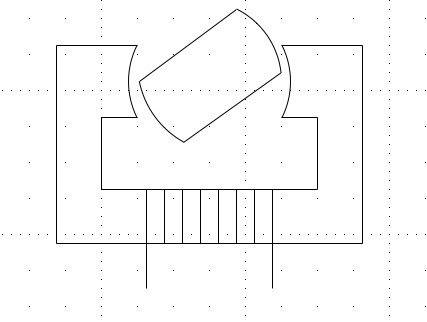# Inductance of a Rotating Machine

AstroFreak

## Homework StatementThe stator of the magnetic circuit of the above figure has a coil of turns 200, which carries a current of 1A. The rotor has a radius of 30mm, a height of 30mm and an axial depth of 20mm. The material for the stator and rotor has infinite permeability. The air gap between the stator and the rotor is 0.1mm and increases rapidly outside the overlap.

a) Determine the max. flux in the air gap and obtain an expression for the inductance of the stator coil as a function of $$\theta$$.

b) Find the max. field energy in each of the air gaps and the corresponding value of $$\theta$$.

c) Calc. the max. torque of that this device can produce.

## Homework Equations

$$Ni = H_c l_c + H_g l_g$$

B = $$\mu_0 H$$

## The Attempt at a Solution

To find the max flux density in the air gap, I use Ampere's Law:

$$Ni = H_c l_c + H_g l_g$$

Since the material of the core has infinite permeability, $$H_c = 0$$

Therefore, $$Ni = H_g l_g$$ and since $$B = \mu_0 H$$

$$Ni = \frac{B}{\mu_0} \times 2 l_g$$

I then arrive at this expression:

$$B = \frac{Ni\mu_0}{2l_g}$$

Sub. the value of $$\mu_0$$ and the length of the air-gap (0.1 mm) and the number of turns (200) and I arrive at a value of 1.26T.

The value seems reasonable to me (i have no answers which I can check against).

The next part of the question has me stumped. How do I calc. the inductance of the machine?

I know that there is going to be an average inductance (lets call that $$L_0$$ and a max. one $$L_{max}$$

The inductance as a function of $$\theta$$ is simply:

$$L(\theta) = L_0 + L_{max}cos(2\theta)$$

To compute the expression, I need to find the average inductance (which is simply $$\frac{L_{max} - L_{min}}{2}$$) and the max one. How can I go about doing this?

Any help?

I could use max. flux density (found above), with flux linkage as

$$\lambda = NBA$$ and $$L = \frac{\lambda}{i}$$

But this only provides me with $$L_{max}$$. How can I go about finding $$L_{min}$$ in order to find the average inductance and hence the expression?